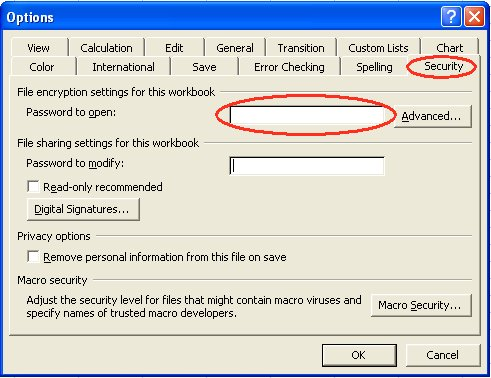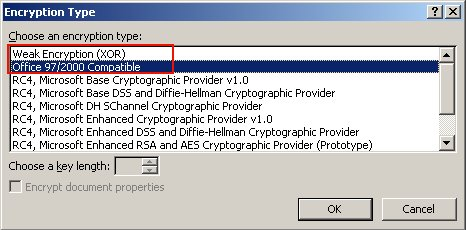# Tracing Precedents and Dependents in Aspose.Cells

Contents
[ ]

## Tracing Precedent and Dependent Cells: Microsoft Excel

Formulas may change based on modifications made by a client. For example, if cell C1 is dependent on C3 and C4 containing a formula, and C1 is changed (so the formula is overriden), C3 and C4, or other cells, need to change to balance the spreadsheet based on business rules.

Similarly, suppose C1 contains the formula “=(B122)/(M2N32)”. I want to find the cells that C1 depends on, that is the precedent cells B1, M2 and N32.

You might need to trace the dependency of a particular cell to other cells. If business rules are embedded in formulas, we would like to find out the dependency and execute some rules based on it. Similarly if the value of a particular cell is modified, which cells in the worksheet are impacted by that change?

Microsoft Excel allows users to trace precedents and dependents.

1. On the View Toolbar, select Formula Auditing. The Formula Auditing dialog is displayed. The Formula Auditing dialog1. Trace Precedents:
1. Select the cell that contains the formula for which you want to find precedent cells.
2. To display a tracer arrow to each cell that directly provides data to the active cell, click Trace Precedents on the Formula Auditing toolbar.
2. Trace formulas that reference a particular cell (dependents)
1. Select the cell for which you want to identify the dependent cells.
2. To display a tracer arrow to each cell that is dependent on the active cell, click Trace Dependents on the Formula Auditing toolbar.

## Tracing Precedent and Dependent Cells: Aspose.Cells

### Tracing Precedents

Aspose.Cells makes it easy to get precedent cells. Not only can it retrieve cells that provide data to a simple formula precedents but also find cells that provide data to a complex formula precedents with named ranges.

In the example below, a template excel file, Book1.xls, is used. The spreadsheet has data and formulas on the first Worksheet.Aspose.Cells provides the Cell class' GetPrecedents method used to trace a cell’s precedents. It returns an ReferredAreaCollection. As you can see above, in Book1.xls, cell B7 contains a formula “=SUM(A1:A3)”. So cells A1:A3 are the precedent cells to cell B7. The following example demonstrates the tracing precedents feature using the template file Book1.xls.

C#

`````` //Instantiating a Workbook object

Workbook workbook = new Workbook("book1.xls");

Cells cells = workbook.Worksheets.Cells;

Aspose.Cells.Cell cell = cells["B7"];

//Tracing precedents of the cell B7.

//The return array contains ranges and cells.

ReferredAreaCollection ret = cell.GetPrecedents();

//Printing all the precedent cells' name.

if(ret != null)

{

for(int m = 0 ; m < ret.Count; m++)

{

ReferredArea area = ret[m];

StringBuilder stringBuilder = new StringBuilder();

{

stringBuilder.Append("[");

stringBuilder.Append(area.ExternalFileName);

stringBuilder.Append("]");

}

stringBuilder.Append(area.SheetName);

stringBuilder.Append("!");

stringBuilder.Append(CellsHelper.CellIndexToName(area.StartRow, area.StartColumn));

if (area.IsArea)

{

stringBuilder.Append(":");

stringBuilder.Append(CellsHelper.CellIndexToName(area.EndRow, area.EndColumn));

}

Console.WriteLine(stringBuilder.ToString());

}

}
``````

### Tracing Dependents

Aspose.Cells lets you get dependent cells in spreadsheets. Aspose.Cells can not only can retrieve cells that provide data regarding a simple formula but also find cells that provide data to a complex formula dependents with named ranges.

Aspose.Cells provides the Cell class' GetDependents method used to trace a cell’s dependents. For example, in Book1.xlsx there are formulas: “=A1+20” and “=A1+30” in the B2 and C2 cells respectively. The following example demonstrates how to trace the dependents for the A1 cell using the template file Book1.xlsx.

C#

`````` string path = "Book1.xlsx";

Workbook workbook = new Workbook(path);

Worksheet worksheet = workbook.Worksheets;

var c = worksheet.Cells["A1"];

var dependents = c.GetDependents(true);

foreach (var dependent in dependents)

{

Debug.WriteLine(string.Format("{0} ---- {1} : {2}", dependent.Worksheet.Name, dependent.Name, dependent.Value));

}
``````